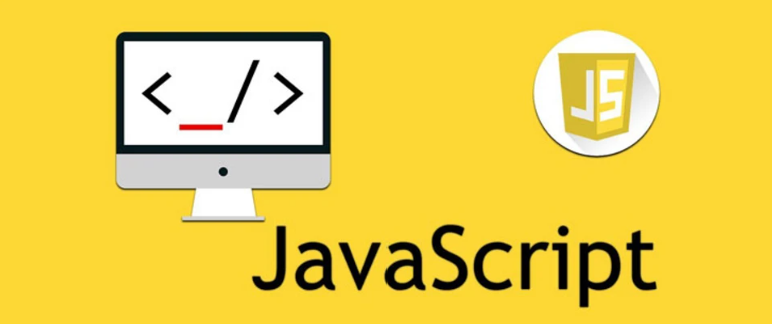# 彻底搞懂JavaScript中的作用域和闭包

deanyuancn

0收藏``````var a = 1;
// 这段代码会被分解为五个词法单元：
var 、 a 、 = 、 1 、 ;``````

``````简单来说，用某种方法可以将
var a = 2;

• 引擎：从头到尾负责整个JavaScript程序的编译及执行过程
• 编译器：负责语法分析及代码生成
• 作用域（今天的主角）：负责收集并维护由所有声明的变量（标识符）注册的一系列查询，并实施一套严格的规则，确定当前执行的代码对这些变量的访问权限。

所以，看似简单的一段代码 var a = 1; 编译器是怎么处理的呢？
``var a = 1;​``

``````function add(a) {
console.log(a + b)
}

var b = 2;

``````function add(a) {
// 对b进行RHS查询 无法找到（未声明）
console.log(a + b) // 对变量b来说，取值操作
b = a // 对变量b来说，赋值操作
}

add(1) // ReferenceError: b is not defined``````
``````function add(a) {
// 对b进行LHS查询，无法找到，会自动创建一个全局变量window.b（非严格模式）
b = a  // 对变量b来说，赋值操作
console.log(a + b)// 对变量b来说，取值操作
}

``````function foo(a) {
var b = a * 2

function bar(c) {
console.log(a, b, c)
}

bar(b * 3)
}

foo(2) // 2, 4, 12``````

foo作用域中有3个变量：a、b、bar
bar作用域中有1个变量：c

``````var a = 1

// 定义一个函数包裹代码块，形成函数作用域
function foo() {
var a = 2
console.log(a) // 2
}

foo()
console.log(a) // 1``````

``````var a = 1;
(function foo() {
var a = 2
console.log(a) // 2
})()
console.log(a) // 1``````

``````for(var i = 0; i < 5; i++) {
console.log(i)
}``````

ES6改变了这种情况，引入let关键字，提供另一种声明变量的方式。

``````{
let a = 2;
console.log(a) // 2
}
console.log(a) // ReferenceError: a is not defined``````

``````for(let i = 0; i < 5; i++) {
console.log(i)
}
console.log(i) // ReferenceError: i is not defined``````

``````{
let j;
for(let j = 0; j < 5; j++) {
let i = j // 每次循环重新赋值
console.log(i)
}
j++
}
console.log(i) // ReferenceError: i is not defined``````

•  提升
先有鸡（声明）还是先有蛋（赋值）？

``````a = 2
var a
console.log(a) // 2

// 引擎解析：
var a
a = 2
console.log(a) // 2``````
``````console.log(a) // undefined
var a = 2

//引擎解析：
var a
console.log(a) // undefined
a = 2``````

``````foo() // 2

var foo = 1

function foo() {
console.log(2)
}

foo = function() {
console.log(3)
}

// 引擎解析：
function foo() {...}
foo()
foo = function() {...}``````

``````foo() // 3

var foo = 1

function foo() {
console.log(2)
}

function foo() {
console.log(3)
}``````

``````foo() // 2

if (true) {
function foo() {
console.log(1)
}
} else {
function foo() {
console.log(2)
}
}``````

``````function foo() {
var a = 2

function bar() {
console.log(a)
}

return bar
}

var baz = foo()
baz() // 2 快看啊，这就是闭包！！！``````

foo()执行后，bar()依然持有该作用域的引用，而这个引用就叫作闭包。

``````for(var i = 0; i < 10; i++) {
setTimeout(function timer() {
console.log(i)
}, i * 1000)
}``````

``````for(var i = 0; i < 10; i++) {
(function(i) {
setTimeout(function timer() {
console.log(i)
}, i * 1000)
})(i)
}``````

``````for(var i = 0; i < 10; i++) {
let j = i // 闭包的块作用域
setTimeout(function timer() {
console.log(j)
}, j * 1000)
}``````

``````for(let i = 0; i < 10; i++) {
setTimeout(function timer() {
console.log(i)
}, i * 1000)
}``````相关问题
社区精华内容